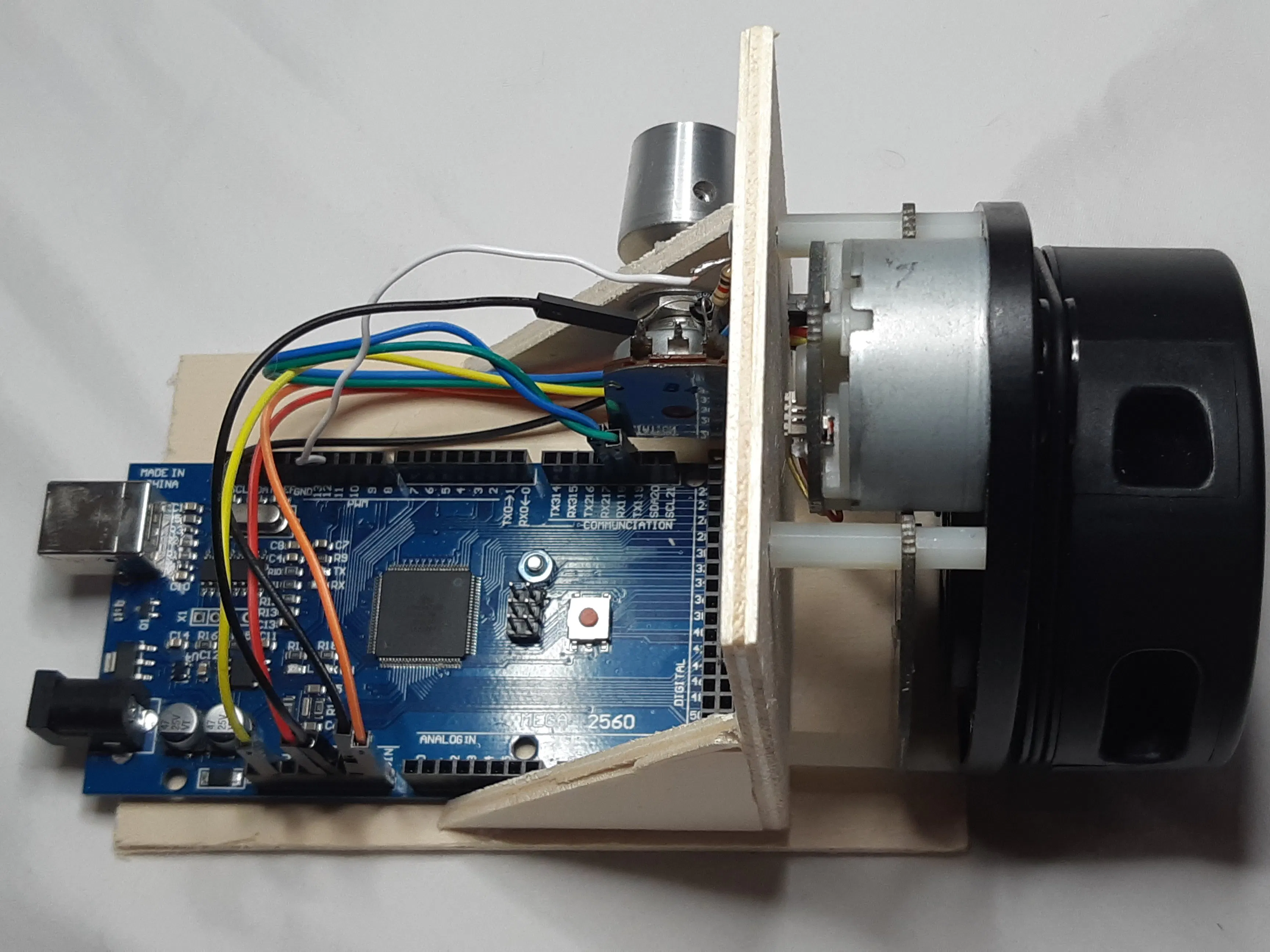# Build a Laser Harp with a LIDAR and Arduino

Let's pick magic chimes out of the air with a LIDAR scanner and an Arduino.

• 10,696 views
• 1 comment
• 25 respects

## About this project

There are many exciting things that you can do with laser. One of these is playing harp! In this project, a LIDAR scanner (LIDAR = Light Detection and Ranging) is used for that. LIDAR works like RADAR, but uses laser instead of microwaves.

The LIDAR sensor used here has a small DC Motor, that drives a turntable with a laser diode and a receiver diode. The Laser diode emits short pulses of Infrared light which are reflected by the neighbored objects around. The reflected light is then detected by the receiver diode. The electronics measures the time between the emission and detection of the reflected light and calculates the distance from the LIDAR. An accuracy of a few millimeters can be achieved with this method!

The LIDAR continuously sends the angle of the laser beam and the measured distance to an Arduino Mega over its UART interface.

The Arduino sketch then extracts the angles of up to two objects (i.e. hands) within a sector of 90 degrees above the scanner and in a distance between 10 cm and 1 meter from the LIDAR. According to these angles, up to two independent chimes are generated.

Instead of simply using the tone() function, the Arduino outputs a PWM signal that represents a sinusoidal wave with a decay. After low-pass-filtering with a 10k resistor and a 1 micro capacitor and amplification with an active speaker, we get a harp-like sound.

Note: An Arduino UNO or similar ATmega328-powered device can also be used here. But in this case, debugging and uploading of sketches is not possible while the LIDAR sensor is connected, because it also uses the UART pins. So, Arduino Mega with its 4 UARTs is more convenient here.

## Code

##### Arduino SketchC/C++
Download and install RPLidar library first: https://github.com/robopeak/rplidar_arduino
```#include <RPLidar.h> //Download from https://github.com/robopeak/rplidar_arduino.git

RPLidar lidar;
#define MOTOR_PIN 3
#define SAMPLE_LEN 156
#define NUM_STRINGS	24
#define OFFSET 36
#define DECAY 20

int16_t samples[SAMPLE_LEN];
const uint16_t max_d = 1000;
const uint16_t min_angle = 135;
const uint16_t max_angle = 225;

int16_t volume1, volume2;
uint32_t cnt1, cnt2; //Index counter

// the setup function runs once when you press reset or power the board
void setup() {
Serial.begin(115200);
for (int i = 0; i < SAMPLE_LEN; i++) {
samples[i] = 10 * sin(20 * PI * i / SAMPLE_LEN);
}
// bind the RPLIDAR driver to the arduino hardware serial
lidar.begin(Serial1);

// set pin modes
pinMode(MOTOR_PIN, OUTPUT);

//PWM Signal generation
DDRB |= (1 << PB5); //OC1A, Pin 11
TCCR1A = (1 << WGM11) + (0 << WGM10);  //Fast PWM 9 Bit
TCCR1B |= (0 << WGM12);
TCCR1A |= (1 << COM1A1); //Toggle OC1A on compare match
TCCR1B = (0 << CS12) + (0 << CS11) + (1 << CS10); //No Prescaling
TIMSK1 = (1 << TOIE1);

//DDRB |= (1 << PB4); //OC2A, Pin 10
//TCCR2A = (1 << COM2A0); //Toggle OC0A on Compare Match
//TCCR2A |= (1 << WGM21) + (0 << WGM20); //CTC
//TCCR2B = (0 << CS22) + (0 << CS21) + (1 << CS20); //Prescaling 256
//TIMSK2 = (1 << OCIE2A);// +(1 << TOIE1);
}

// the loop function runs over and over again until power down or reset
float freq_1, freq_2;
int16_t string1, string2;
float freq;
void loop(){
uint16_t pause;
static long t1, t2;
//return;
if (IS_OK(lidar.waitPoint())) {
float d = lidar.getCurrentPoint().distance; //distance value in mm unit
float angle = lidar.getCurrentPoint().angle; //anglue value in degree
bool  startBit = lidar.getCurrentPoint().startBit; //whether this point belongs to a new scan
byte  quality = lidar.getCurrentPoint().quality; //quality of the current measurement
if (quality && (angle >= min_angle) && (angle <= max_angle) && (d < max_d)) {
if(!string1)
string1 = NUM_STRINGS * (angle - min_angle) / (max_angle - min_angle);
if (string1) {
string2 = NUM_STRINGS * (angle - min_angle) / (max_angle - min_angle);
if (abs(string2 - string1) <= 2) //Don't play adjacent strings. Sound odd
string2 = 0;
}
}

if (startBit) {
if (string1 != 0) {
freq_1 = 0.44 * pow(2.0, ((float)string1 + OFFSET - 49) / 12);
volume1 = 10;
}
else if (volume1 > 0){
volume1--;
delay(DECAY);
}
string1 = 0;

if (string2 != 0) {
freq_2 = 0.44 * pow(2.0, ((float)string2 + OFFSET - 49) / 12);
volume2 = 10;
}
else if (volume2 > 0) {
volume2--;
delay(DECAY);
}
string2 = 0;
}
}
else {
digitalWrite(MOTOR_PIN, 0); //stop the rplidar motor
Serial.println("Stop Motor");

// try to detect RPLIDAR...
rplidar_response_device_info_t info;
if (IS_OK(lidar.getDeviceInfo(info, 100))) {
Serial.println("Lidar detected...");
lidar.startScan();

// start motor rotating at max allowed speed
digitalWrite(MOTOR_PIN, 1);
delay(1000);
}
else {
Serial.println("Lidar Error");
}
}
}

ISR(TIMER1_OVF_vect) {
cnt1++;
if(cnt1 % 2 == 0)
OCR1A = 256 + volume1 * samples[(uint16_t)(cnt1 * freq_1) % SAMPLE_LEN];
else
OCR1A = 256 + volume2 * samples[(uint16_t)(cnt1 * freq_2) % SAMPLE_LEN];
}
```

## Comments

#### UW-Makeathon: Laser Drums

Project in progress by Team Laser Drums

• 3,084 views
• 0 comments
• 6 respects

• 8,736 views
• 6 comments
• 9 respects

#### Laser Guitar

Project tutorial by yousseftekriti and samnaji

• 2,431 views
• 1 comment
• 8 respects

• 19,828 views
• 12 comments
• 53 respects

#### Laser Pointer Panther Tank

Project tutorial by Arduino “having11” Guy

• 8,866 views
• 1 comment
• 16 respects

#### Kravox! Wireless, Motion and Touch-Sensing Instrument

Project tutorial by Tim Krahmer

• 11,528 views
• 20 comments
• 37 respects